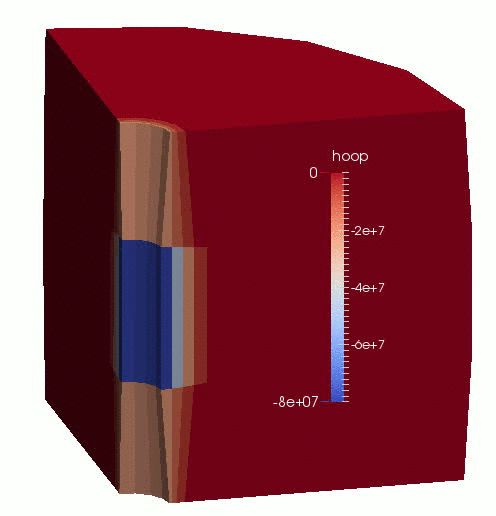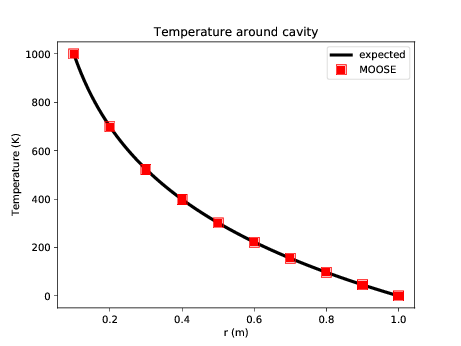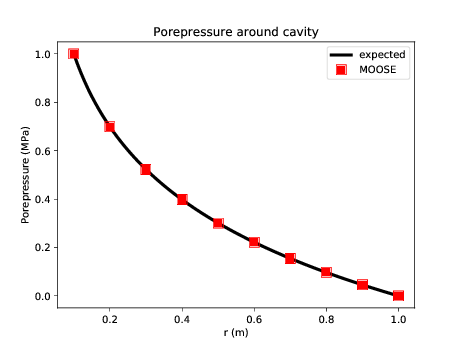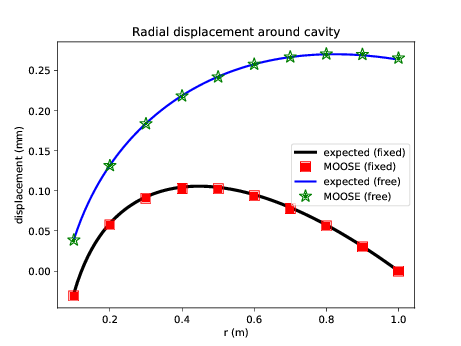# Porous Flow Tutorial Page 04. Adding solid mechanics

In this Page, solid mechanics is added to the thermo-hydro simulation of preious Pages. The equations are discussed in governing equations. Only quasi-static solid mechanics is considered here, without gravity, so the equations read (1) (2) (3) As described previously, is the porepressure, the temperature and is the Biot coefficient. The additional nomenclature used here is

• is the effective stress tensor

• is the total stress tensor

• is the elasticity tensor of the drained porous skeleton

• is the linear thermal expansion coefficient. Note that this is the linear version, in contrast to the volumetric coefficients introduced in Page 1.

Once again, before attempting to write an input file, a rough estimate of the expected nonlinear residuals must be performed, as discussed in convergence criteria. The residual for the Eq. (3) is approximately (4) Corresponding to the choice Pa.m made in Page 02 the choice Pa.m may be made here. This means which is significantly greater than for the fluid equation. Therefore, the displacement variables are scaled by .

Many mechanically-related MOOSE objects (Kernels, BCs, etc) accept the use_displaced_mesh input parameter. For virtually all PorousFlow simulations, it is appropriate to set this to false: use_displaced_mesh = false. This means that the Kernel's residual (or BC's residual, Postprocessor's value, etc) will be evaluated using the undisplaced mesh. This has the great numerical advantage that the solid-mechanics elasticity equations remain linear.

Also, many mechanically-related MOOSE objects require the displacements input parameter. Therefore, it is convenient to put this parameter into the GlobalParams block:

[GlobalParams]
displacements = 'disp_x disp_y disp_z'
PorousFlowDictator = dictator
biot_coefficient = 1.0
[]

(modules/porous_flow/examples/tutorial/04.i)

To model this thermo-hydro-mechanical system, the PorousFlowBasicTHM action needs to be enhanced to read:

[Variables]
[./porepressure]
[../]
[./temperature]
initial_condition = 293
scaling = 1E-8
[../]
[./disp_x]
scaling = 1E-10
[../]
[./disp_y]
scaling = 1E-10
[../]
[./disp_z]
scaling = 1E-10
[../]
[]

[PorousFlowBasicTHM]
porepressure = porepressure
temperature = temperature
coupling_type = ThermoHydroMechanical
gravity = '0 0 0'
fp = the_simple_fluid
thermal_eigenstrain_name = thermal_contribution
use_displaced_mesh = false
[]

(modules/porous_flow/examples/tutorial/04.i)

The boundary conditions used here are roller boundary conditions, as well as boundary conditions that model the effect of the fluid porepressure on the injection area:

  [./roller_tmax]
type = PresetBC
variable = disp_x
value = 0
boundary = tmax
[../]
[./roller_tmin]
type = PresetBC
variable = disp_y
value = 0
boundary = tmin
[../]
[./roller_top_bottom]
type = PresetBC
variable = disp_z
value = 0
boundary = 'top bottom'
[../]
[./cavity_pressure_x]
type = Pressure
boundary = injection_area
variable = disp_x
component = 0
factor = 1E6
use_displaced_mesh = false
[../]
[./cavity_pressure_y]
type = Pressure
boundary = injection_area
variable = disp_y
component = 1
factor = 1E6
use_displaced_mesh = false
[../]

(modules/porous_flow/examples/tutorial/04.i)

The TensorMechanics module of MOOSE provides some useful AuxKernels for extracting effective stresses of interest to this problem (the effective radial stress and the effective hoop stress)

[AuxVariables]
[./stress_rr]
family = MONOMIAL
order = CONSTANT
[../]
[./stress_pp]
family = MONOMIAL
order = CONSTANT
[../]
[]

[AuxKernels]
[./stress_rr]
type = RankTwoScalarAux
rank_two_tensor = stress
variable = stress_rr
point1 = '0 0 0'
point2 = '0 0 1'
[../]
[./stress_pp]
type = RankTwoScalarAux
rank_two_tensor = stress
variable = stress_pp
scalar_type = HoopStress
point1 = '0 0 0'
point2 = '0 0 1'
[../]
[]

(modules/porous_flow/examples/tutorial/04.i)

Finally, some mechanics-related Materials need to be defined

  [./elasticity_tensor]
type = ComputeIsotropicElasticityTensor
youngs_modulus = 5E9
poissons_ratio = 0.0
[../]
[./strain]
type = ComputeSmallStrain
eigenstrain_names = thermal_contribution
[../]
[./thermal_contribution]
type = ComputeThermalExpansionEigenstrain
temperature = temperature
thermal_expansion_coeff = 0.001 # this is the linear thermal expansion coefficient
eigenstrain_name = thermal_contribution
stress_free_temperature = 293
[../]
[./stress]
type = ComputeLinearElasticStress
[../]

(modules/porous_flow/examples/tutorial/04.i)

An animation of the results is shown in Figure 1.Figure 1: Displacement (magnified by 100 times) and effective hoop-stress evolution in the borehole-aquifer-caprock system.

The dynamics of this model are fascinating, and readers are encouraged to pause and play with parameters to explore how they effect the final result. In fact, this model is very similar to the "THM Rehbinder" test in PorousFlow's test suite. Rehbinder (Rehbinder, 1995) derived analytical solutions for a similar THM problem, and MOOSE replicates his result exactly:Figure 2: Comparison between MOOSE and Rehbinder's analytical solution.Figure 3: Comparison between MOOSE and Rehbinder's analytical solution.Figure 4: Comparison between MOOSE and Rehbinder's analytical solution.

1. G. Rehbinder. Analytical solutions of stationary coupled thermo-hydro-mechanical solutions. Int J Rock Mech Min Sci and Geomech Abstr, 32:453–463, 1995.[BibTeX]